Question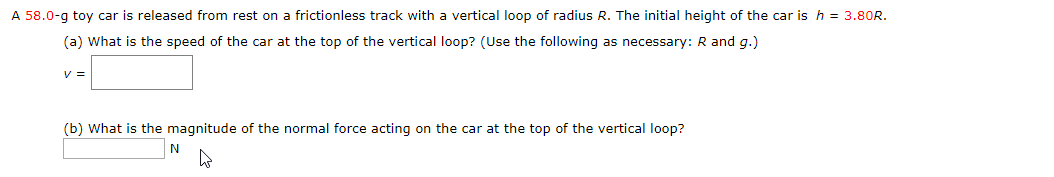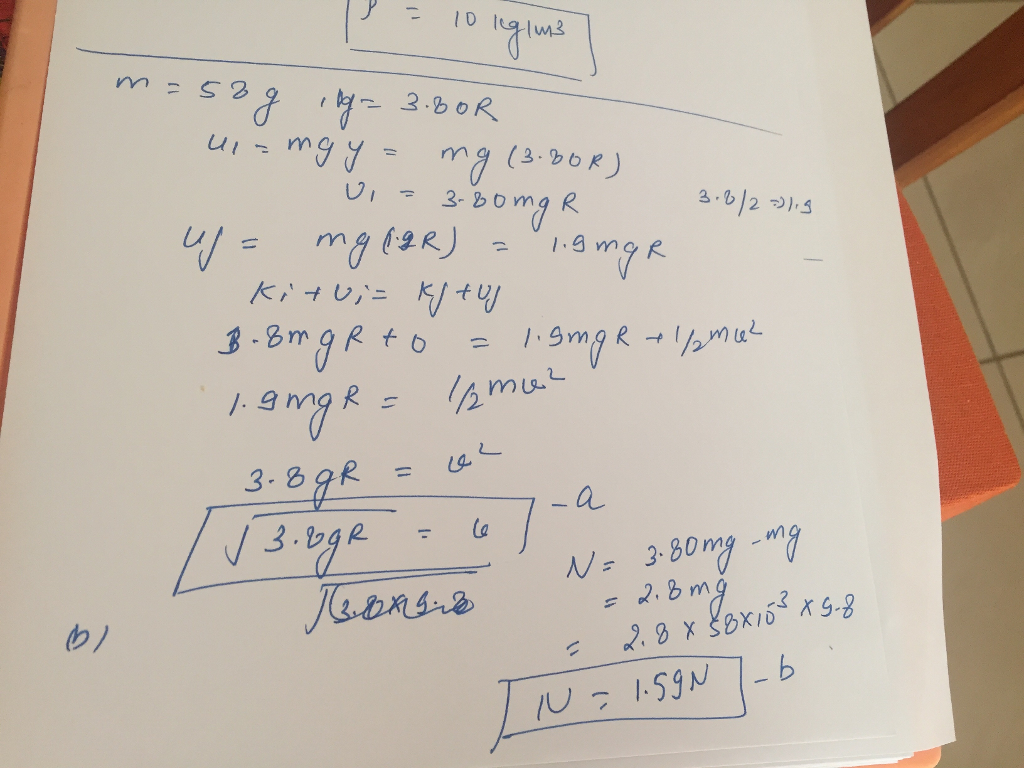#### Earn Coins

Coins can be redeemed for fabulous gifts.

Similar Homework Help Questions
• ### A 48.5-g toy car is released from rest on a frictionless track with a vertical loop...

A 48.5-g toy car is released from rest on a frictionless track with a vertical loop of radius R. The initial height of the car is h = 4.45R. (a) What is the speed of the car at the top of the vertical loop? (Use the following as necessary: R and g.) (b) What is the magnitude of the normal force acting on the car at the top of the vertical loop?

• ### A small block of mass m slides along the frictionless loop the loop track shown below....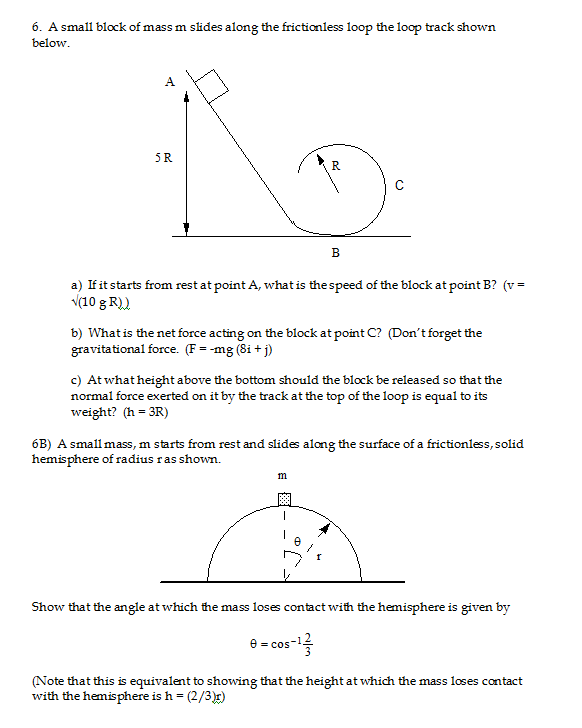A small block of mass m slides along the frictionless loop the loop track shown below. If it starts from rest at point A, what is the speed of the block at point B? (v = squareroot (10 g R)) What is the net force acting on the block at point C? (Don't forget the gravitational force. (F = -mg (8i + j) At what height above the bottom should the block be released so that the normal force exerted...

• ### A roller coaster car of mass 800 kg when released from rest at point A (height...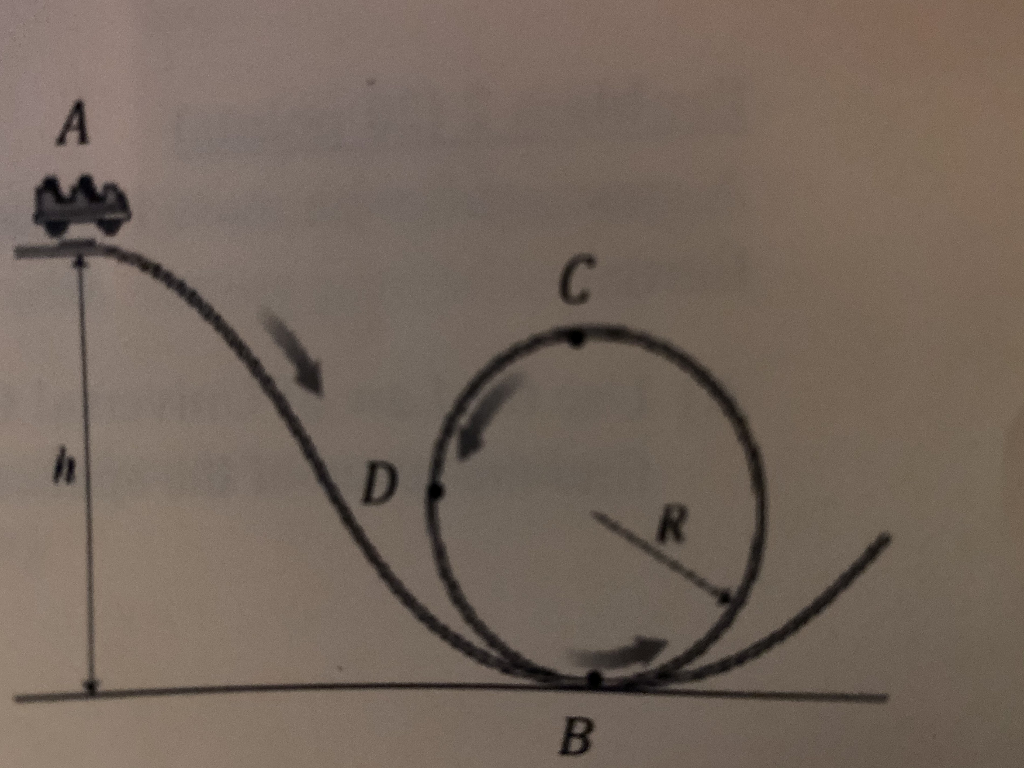A roller coaster car of mass 800 kg when released from rest at point A (height h above the ground) slides along the track and inside the loop of radius 16.0 m. The car never loses contact with the track. A) Draw below a free body diagram for the car the car at the top of the loop. B) If the normal force acting on the car at the top is 1500 N, what is the speed of the car...

• ### A roller coaster car of mass 800 kg when released from rest at point A (height...A roller coaster car of mass 800 kg when released from rest at point A (height h above the ground) slides along the track and inside the loop of radius 16.0 m. The car never loses contact with the track. A) Draw below a free body diagram for the car the car at the top of the loop. B) If the normal force acting on the car at the top is 1500 N, what is the speed of the car...

• ### A piece of soap of mass m, initially at rest, is released onto a slippery track...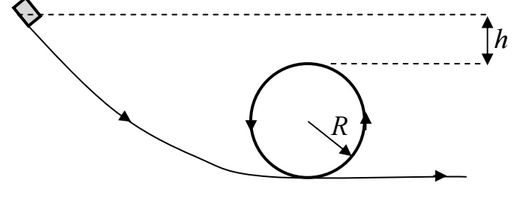A piece of soap of mass m, initially at rest, is released onto a slippery track from a height h = R/2 above the top of a loop of radius R (see diagram below). What is the magnitude of the normal force acting on the soap as it begins its circular trajectory at the base of the loop?

• ### A toy car is released at the top of a frictionless track as shown in the...

A toy car is released at the top of a frictionless track as shown in the illustration. Rank the magnitude of the velocity of the car at positions A, B, and C from greatest to least.

• ### In a loop-the-loop ride a car goes around a vertical, circular loop at a constant speed....

In a loop-the-loop ride a car goes around a vertical, circular loop at a constant speed. The car has a mass m = 286 kg and moves with speed v = 13.82 m/s. The loop-the-loop has a radius of R = 8 m. 1) What is the magnitude of the normal force on the care when it is at the bottom of the circle? (But as the car is accelerating upward.) 2) What is the magnitude of the normal force...

• ### In a loop-the-loop ride a car goes around a vertical, circular loop at a constant speed....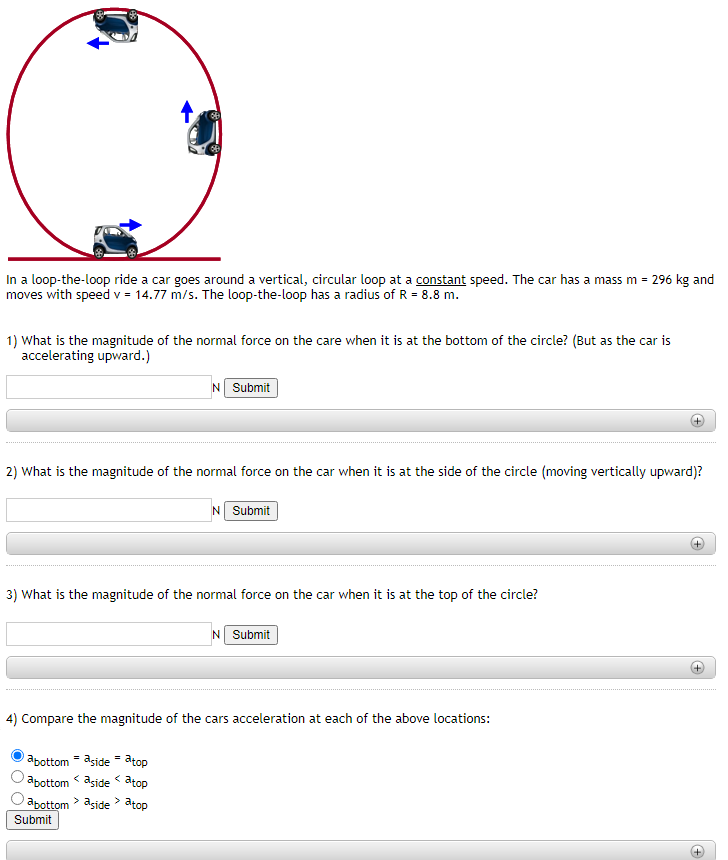In a loop-the-loop ride a car goes around a vertical, circular loop at a constant speed. The car has a mass m = 296 kg and moves with speed v = 14.77 m/s. The loop-the-loop has a radius of R = 8.8 m. 1) What is the magnitude of the normal force on the care when it is at the bottom of the circle? (But as the car is accelerating upward.) N Submit 2) What is the magnitude of the...

• ### 21. A frictionless roller coaster car is released from rest on the track at a height...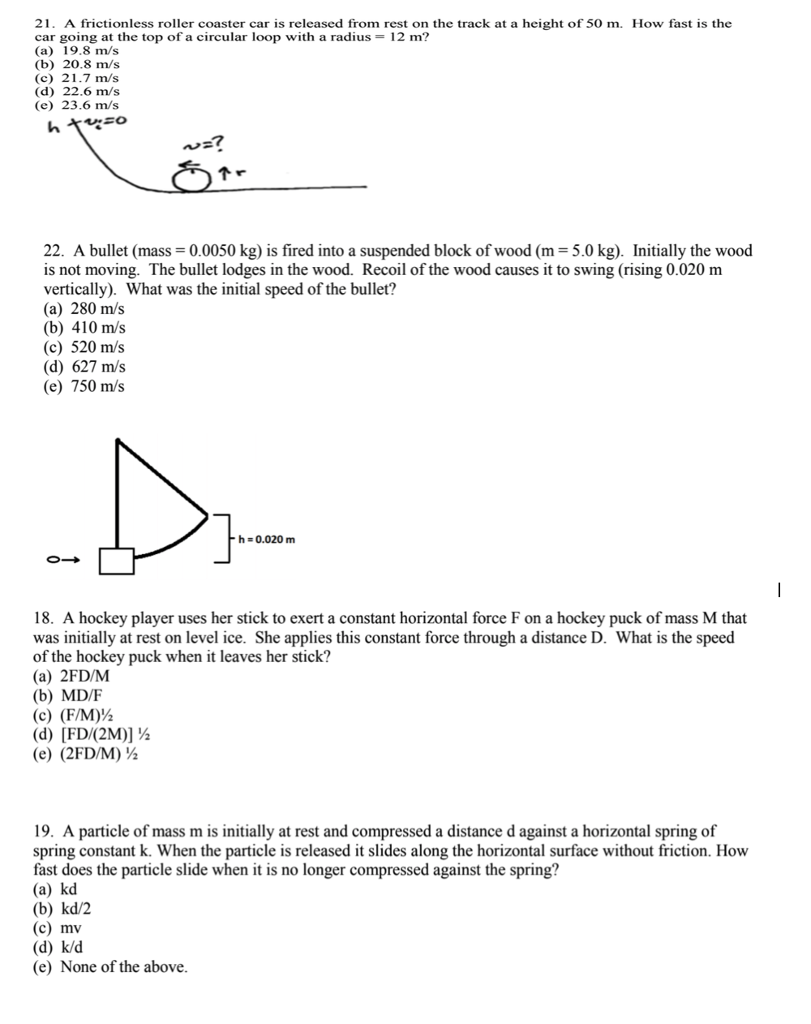21. A frictionless roller coaster car is released from rest on the track at a height of 50 m. How fast is the car going at the top of a circular loop with a radius- 12 m? (a) 19.8 m/s (b) 20.8 m/s (c) 21.7 m/s (d) 22.6 m/s (e) 23.6 m/s 7 22. A bullet (mass-0.0050 kg) is fired into a suspended block of wood (m-5.0 kg). Initially the wood is not moving. The bullet lodges in the wood....

• ### A student with mass 58.0 kg rides on a roller coaster with a vertical loop. At...

A student with mass 58.0 kg rides on a roller coaster with a vertical loop. At the top of the loop the track has a radius of 19.7 m and the roller coaster moves at speed of 21.9 m/s at the top. Determine the normal force from the seat on the student at the top of the loop. Give your answer in newtons (N) and with 3 significant figures.